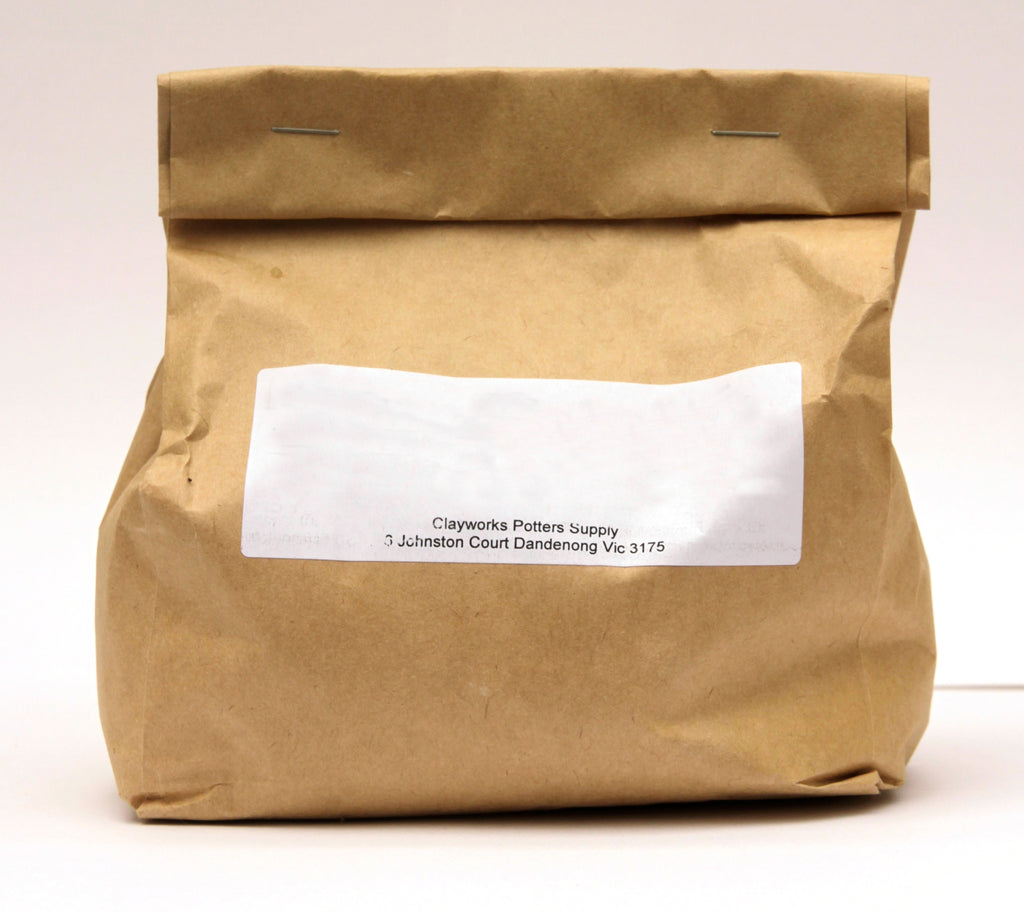# Soda Feldspar

\$10.60

Chemical analysis

Silicon dioxide greater than or equal to 70%

Aluminium dioxide greater than or equal to 16%

Sodium oxide greater than or equal to 9%

Iron oxide less than or equal to 0.5%

Titanium dioxide less than or equal to 0.5%

Calcium Oxide less than or equal to 1%

Potassium oxide less than or equal to .5%

Loss on ignition less than or equal to 1%